#### Testing for randomness

Thus, it is important to realize that even the random'' samples are deterministic. They are designed to optimize performance on statistical tests. Many sophisticated statistical tests of uniform randomness are used. One of the simplest, the chi-square test, is described here. This test measures how far computed statistics are from their expected value. As a simple example, suppose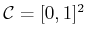and is partitioned into a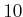byarray of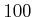square boxes. If a setofsamples is chosen at random, then intuitively each box should receive roughly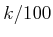of the samples. An error function can be defined to measure how far from true this intuition is: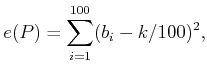(5.18)

in which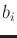is the number of samples that fall into box. It is shown  that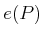follows a chi-squared distribution. A surprising fact is that the goal is not to minimize. If the error is too small, we would declare that the samples are too uniform to be random! Imagine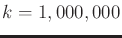and exactly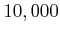samples appear in each of theboxes. This yields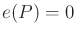, but how likely is this to ever occur? The error must generally be larger (it appears in many statistical tables) to account for the irregularity due to randomness.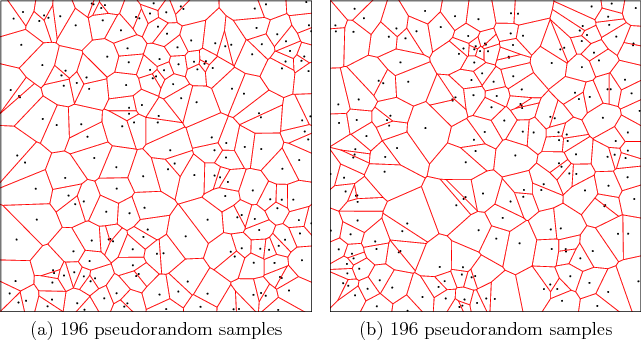This irregularity can be observed in terms of Voronoi diagrams, as shown in Figure 5.3. The Voronoi diagram partitions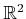into regions based on the samples. Each samplehas an associated Voronoi region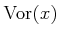. For any point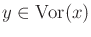,is the closest sample to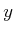using Euclidean distance. The different sizes and shapes of these regions give some indication of the required irregularity of random sampling. This irregularity may be undesirable for sampling-based motion planning and is somewhat repaired by the deterministic sampling methods of Sections 5.2.3 and 5.2.4 (however, these methods also have drawbacks).

Steven M LaValle 2020-08-14